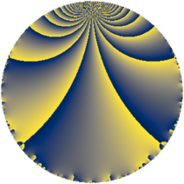# Properties

 Label 72.2.aLevel $72$ Weight $2$ Character orbit 72.a Rep. character $\chi_{72}(1,\cdot)$ Character field $\Q$ Dimension $1$ Newform subspaces $1$ Sturm bound $24$ Trace bound $0$

# Related objects

## Defining parameters

 Level: $$N$$ $$=$$ $$72 = 2^{3} \cdot 3^{2}$$ Weight: $$k$$ $$=$$ $$2$$ Character orbit: $$[\chi]$$ $$=$$ 72.a (trivial) Character field: $$\Q$$ Newform subspaces: $$1$$ Sturm bound: $$24$$ Trace bound: $$0$$

## Dimensions

The following table gives the dimensions of various subspaces of $$M_{2}(\Gamma_0(72))$$.

Total New Old
Modular forms 20 1 19
Cusp forms 5 1 4
Eisenstein series 15 0 15

The following table gives the dimensions of the cuspidal new subspaces with specified eigenvalues for the Atkin-Lehner operators and the Fricke involution.

$$2$$$$3$$FrickeDim.
$$+$$$$-$$$$-$$$$1$$
Plus space$$+$$$$0$$
Minus space$$-$$$$1$$

## Trace form

 $$q + 2 q^{5} + O(q^{10})$$ $$q + 2 q^{5} - 4 q^{11} - 2 q^{13} - 2 q^{17} - 4 q^{19} + 8 q^{23} - q^{25} - 6 q^{29} + 8 q^{31} + 6 q^{37} + 6 q^{41} + 4 q^{43} - 7 q^{49} + 2 q^{53} - 8 q^{55} - 4 q^{59} - 2 q^{61} - 4 q^{65} - 4 q^{67} - 8 q^{71} + 10 q^{73} - 8 q^{79} + 4 q^{83} - 4 q^{85} + 6 q^{89} - 8 q^{95} + 2 q^{97} + O(q^{100})$$

## Decomposition of $$S_{2}^{\mathrm{new}}(\Gamma_0(72))$$ into newform subspaces

Label Dim $A$ Field CM Traces A-L signs $q$-expansion
$a_{2}$ $a_{3}$ $a_{5}$ $a_{7}$ 2 3
72.2.a.a $1$ $0.575$ $$\Q$$ None $$0$$ $$0$$ $$2$$ $$0$$ $+$ $-$ $$q+2q^{5}-4q^{11}-2q^{13}-2q^{17}-4q^{19}+\cdots$$

## Decomposition of $$S_{2}^{\mathrm{old}}(\Gamma_0(72))$$ into lower level spaces

$$S_{2}^{\mathrm{old}}(\Gamma_0(72)) \cong$$ $$S_{2}^{\mathrm{new}}(\Gamma_0(24))$$$$^{\oplus 2}$$$$\oplus$$$$S_{2}^{\mathrm{new}}(\Gamma_0(36))$$$$^{\oplus 2}$$